## Electric Power System (Model of Dobson and Chiang)

Original source of the model: I. Dobson, H.-D. Chiang: Towards a Theory of Voltage Collapse in Electric Power Systems. Systems and Control Letters 13 (1989) 253-262.

The example exhibits a turning point, a Hopf bifurcation, and a period doubling sequence. All of the bifurcations are essential ingredients of a risk analysis for electric power systems. Figures 15 and 16 show a simulation for varying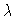(the reactive power demand). The simulation illustrates a collapse of the voltage V of a generator.

For the other figures, the parameter is kept (quasi-)stationary. Figure 1 and 2 show the bifurcation scenario, the other figures show phase portraits and time history for a selection of parameter values. The development of a blue-sky catastrophe is illustrated in Figures 3-5. Figures 10-14 report on the growing complexity of the orbits whenis passing through the range of period doubling. This leads to chaos, which is illustrated in Figure 9.

For further reference on the risk aspect, see R. Seydel: Assessing Voltage Collapse. Proceedings of a Conference on Risk Analysis. Paris 1998.

Figure 1
Bifurcation diagram V versus load(detail: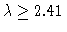, all stationary states and some periodic states; for the latter ones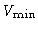is depicted).

Figure 2
Bifurcation diagram V andversus load. detail: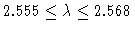, all stationary states and some periodic states (S: stationary, P: periodic, s: stable, u: unstable, HB: Hopf bifurcation, PD: periodic doubling)

Figure 3
Phase diagram (projection). Horizontal axis: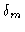; vertical axis: V. (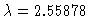)

Figure 4
Phase diagram (projection). Horizontal axis:; vertical axis: V. (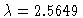)

Figure 5
Phase diagram (projection). Horizontal axis:; vertical axis: V. (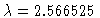)

Figure 6
Phase diagram, periodic orbit, unstable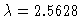, T=4.6376, projection: horizontal axis, vertical axis V.

Figure 7
Phase diagram, two periodic orbits for, one stable (T=3.802) and one unstable (T=1.913) projection: horizontal axis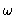, vertical axis V.

Figure 8
Phase diagram, unstable periodic orbit,, T=7.62, projection: horizontal axis, vertical axis V.

Figure 9
Phase diagram,,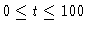. projection: horizontal axis, vertical axis V.

Figure 10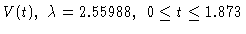. Periodic orbit: simple'' period.

Figure 11. Periodic orbit: double'' period.

Figure 12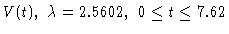. Periodic orbit: fourfold'' period.

Figure 13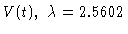Figure 14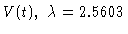Figure 15
V(t), withvarying linearly from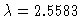to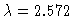. Collapse of voltage.

Figure 16
Phase diagram corresponding to previous figure. Projection to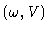-plane,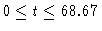, withvarying linearly fromto.

This Example
Figure 1
Figure 2
Figure 3
Figure 4
Figure 5
Figure 6
Figure 7
Figure 8
Figure 9
Figure 10
Figure 11
Figure 12
Figure 13
Figure 14
Figure 15
Figure 16